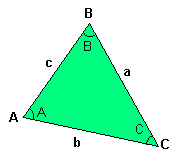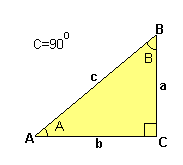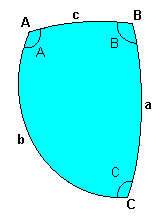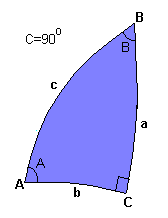### TRIGONOMETRY

Trigonometry is the branch of mathematics that deals with the relationships between the sides and angles of triangles.

PLANE TRIGONOMETRY

Plane trigonometry is the trigonometry of triangles in a plane (ie 2D).Scalene triangle in a plane

This triangle is defined by three connected line segments. The vertices are labelled A, B and C, and these symbols are also used to label the interior angles at these vertices. The sides opposite each angle have a linear dimension labelled by the corresponding lowercase letter (ie side a is opposite angle A). The three interior angles sum to 180 degrees:

A + B + C = 180o
There are two fundamental laws relating the sides and angles:
The law of sines
a / sin A = b / sin B = c / sin C

The law of cosines
c2 = a2 + b2 - 2 a b cos C

When one of the angles (eg C) is equal to 90 degrees, we have a right-angled triangle.Planar right triangle

The above two laws then essentially reduce to basic definitions of the fundamental trigonometrical functions:

sin A = a / c
cos A = sin( 90o - A ) = sin B = b / c
[ tan A = sin A / cos A = a / b ]

and the Pythagorean relation
c2 = a2 + b2

SPHERICAL TRIGONOMETRY

Spherical trigonometry is the trigonometry of triangles on the surface of a sphere (ie 3-D)General triangle on the surface of a sphere

A spherical triangle is a triangle existing on the surface of a sphere, defined by line segments joining three points A, B and C. The line segments are the shortest distances on the sphere between their respective end points, and as a result are portions of great circles with their centres at the centre of the sphere.

As with plane trigonometry, the symbols A, B and C are also used to label the angles between the line segments (or the planes containing the line segments) on the surface of the sphere. However, unlike plane trigonometry the sides a, b and c are not linear distances, but angles. These angles are the angles subtended by the respective line segments at the center of the sphere. In a spherical triangle the angles A, B and C do not sum to 180 degrees.

There are also two fundamental laws of spherical trigonometry:

The law of sines
sin a / sin A = sin b / sin B = sin c / sin C

The law of cosines
cos c = cos a cos b + sin a sin b cos C

When one of the included angles (eg C) is equal to 90 degrees, we have a spherical right triangle.Spherical triangle with one right angle

The law of sines then reduces to:

sin a = sin A sin c
sin b = sin B sin c
and the law of cosines reduces to:
cos c = cos a cos b

APPLICATIONS

Both plane and spherical trigonometry play an essential role in the solution of many problems involving astronomy, space navigation, geodesy, surveillance, spacecraft design and planetary science.